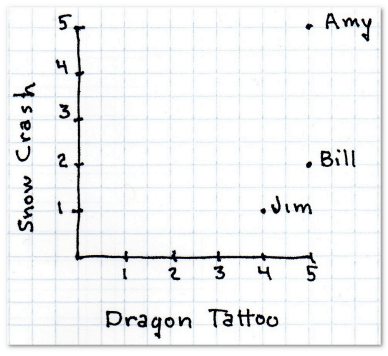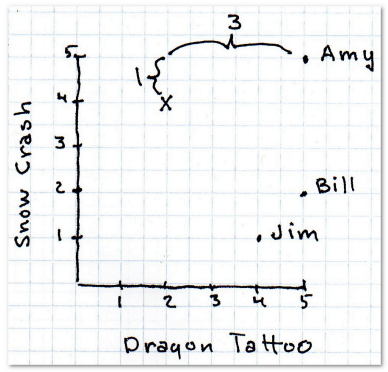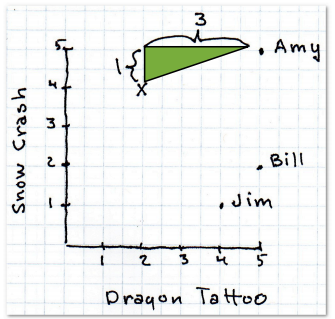@guoxs 2016-05-21T06:06:15.000000Z 字数 2441 阅读 1978

# 推荐系统入门

数据挖掘## 曼哈顿距离def manhattan(rating1, rating2):    """计算曼哈顿距离。rating1和rating2参数中存储的数据格式均为    {'The Strokes': 3.0, 'Slightly Stoopid': 2.5}"""    distance = 0    for key in rating1:        if key in rating2:            distance += abs(rating1[key] - rating2[key])    return distance

## 欧几里得距离• r = 1 该公式即曼哈顿距离
• r = 2 该公式即欧几里得距离
• r = ∞ 极大距离

r值越大，单个维度的差值大小会对整体距离有更大的影响。

def minkowski(rating1, rating2, r):    distance = 0    for key in rating1:        if key in rating2:            distance += pow(abs(rating1[key] - rating2[key]), r)    return pow(distance, 1.0 / r)# 修改computeNearestNeighbor函数中的一行distance = minkowski(users[user], users[username], 2)# 这里2表示使用欧几里得距离

## 皮尔逊相关系数

from math import sqrtdef pearson(rating1, rating2):    sum_xy = 0    sum_x = 0    sum_y = 0    sum_x2 = 0    sum_y2 = 0    n = 0    for key in rating1:        if key in rating2:            n += 1            x = rating1[key]            y = rating2[key]            sum_xy += x * y            sum_x += x            sum_y += y            sum_x2 += pow(x, 2)            sum_y2 += pow(y, 2)    # 计算分母    denominator = sqrt(sum_x2 - pow(sum_x, 2) / n) * sqrt(sum_y2 - pow(sum_y, 2) / n)    if denominator == 0:        return 0    else:        return (sum_xy - (sum_x * sum_y) / n) / denominator

## 选择何种相似度？

• 如果数据存在“分数膨胀”问题，就使用皮尔逊相关系数。
• 如果数据比较“密集”，变量之间基本都存在公有值，且这些距离数据是非常重要的，那就使用欧几里得或曼哈顿距离。
• 如果数据是稀疏的，则使用余弦相似度。

## K最邻近算法• 私有
• 公开
• 删除